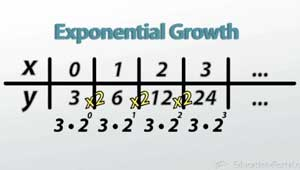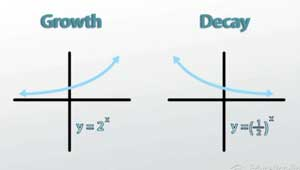# Exponential Growth vs. Decay

An error occurred trying to load this video.

Try refreshing the page, or contact customer support.

Coming up next: What is a Logarithm?

### You're on a roll. Keep up the good work!

Replay
Your next lesson will play in 10 seconds
• 0:13 Exponential Growth
• 1:13 Exponential Decay
• 4:05 Growth vs. Decay
• 5:39 Determining a Starting Value
• 8:02 Lesson Summary

Want to watch this again later?

Timeline
Autoplay
Autoplay
Speed

#### Recommended Lessons and Courses for You

Lesson Transcript
Instructor: Eric Garneau
How is it that it only takes four years for our computer to go from top-of-the-line to almost worthless? Well, it has something to do with what's called exponential decay, which we'll learn about here!

## Exponential GrowthWe know that an exponential function is any pattern of numbers that is continuously multiplied by something - for example 3, 6 (3*2), 12 (3*4), 24 (3*8). This is an exponential function because I start with a number 3 and continuously multiply by 2. We can represent this with an equation by saying that y = 3*2x, because the pattern starts at 3 and then is multiplied by 2 each step of the way. If you substitute in x values starting at 0 and remembering that 0 exponents equal 1, you'll find that we get this exact same pattern. This example is what is called exponential growth because the numbers are growing exponentially, but there is another type of exponential function whose entries get smaller instead of getting bigger, exponential decay.

## Exponential Decay

Exponential decay still follows the same rule of repeated multiplication, only the number we multiply by has to be smaller than one. What this ends up looking like is something that appears to be repeated division. Let's take a look at an example that most of us can relate to, our computer becoming worthless way faster than we had expected.Say you buy a pretty nice new computer for \$1,600, but after you've owned it for a year, it's already worth only 1/2 as much as it used to be. That makes it worth \$800 after 1 year, and if this pattern continues it would be worth \$400 after 2 years, \$200 after 3, and only \$100 after a short 4 years. The price of your computer is 'decaying' (or getting smaller) each year by a factor of 1/2. It kind of looks like division by 2, but remember that division by 2 is the same as multiplication by 1/2. That means that because your pattern begins at 1,600 and becomes repeatedly multiplied by 1/2, the exponential decay equation for this example would be y = 1,600*(1/2)x.

These exponential functions get really small, really fast. If we assume this pattern continues, your computer would only be worth \$1.50 after ten years! But it's important to note that no matter how long you keep your computer, it will never be worth \$0. It might seem like it, and it might be worth pretty close to zero, but multiplying by 1/2 over and over can only make a number that's really really small. Maybe you'll end up with 0.0000000000000000001, but it will always have that little 1 on the end. If I divide this by 2, which is smaller, now it's 0.00000000000000000005. There's still a number down there; no matter how many times I divide by two, it's still going to be a tiny bit bigger than zero. This means that on our graph our line is going to get closer and closer and closer to the x-axis but it'll never quite get there. This is a math concept that comes up pretty often and is called an asymptote. In this case we have an asymptote at y = 0 because that is the line (which is basically the x-axis) that our graph will get infinitely close to but never quite touch.

## Growth vs. DecayTo unlock this lesson you must be a Study.com Member.

### Register to view this lesson

Are you a student or a teacher?

### Unlock Your Education

#### See for yourself why 30 million people use Study.com

##### Become a Study.com member and start learning now.
Back
What teachers are saying about Study.com

### Earning College Credit

Did you know… We have over 160 college courses that prepare you to earn credit by exam that is accepted by over 1,500 colleges and universities. You can test out of the first two years of college and save thousands off your degree. Anyone can earn credit-by-exam regardless of age or education level.